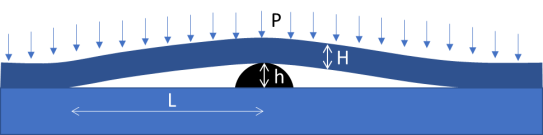## Defect Zones

### Quick Start

Imagine a 5μm particle trapped between two surfaces being pushed together in, say, a lamination. Obviously it stops contact at that point. But how far does the absence of contact extend, i.e. what is the size of the defect zone? The answer is "surprisingly large".

### Credits

The app is based on an analysis created by Dr Dilwyn Jones.

### Defect Zones

Modulus E GPa
Thickness h μm
Defect r μm
Pressure P MPa
Stiffness S μN.m
L μm
//One universal basic required here to get things going once loaded
Main();
};
//Any global variables go here

//Main is hard wired as THE place to start calculating when input changes
//It does no calculations itself, it merely sets them up, sends off variables, gets results and, if necessary, plots them.
function Main() {
saveSettings();

//Send all the inputs as a structured object
//If you need to convert to, say, SI units, do it here!
const inputs = {
E: sliders.SlideE.value * 1e9, //From GPa
P: sliders.SlideP.value * 1e6, //From MPa
h: sliders.Slideh.value * 1e-6, //From um
r: sliders.Slider.value * 1e-6, //From um
}

//Get all the resonses as a structure
const result = CalcIt(inputs)

//Set all the text box outputs
document.getElementById('S').value = result.S
document.getElementById('L').value = result.L
}

//Here's the real app calculation
function CalcIt({ E, P,h,r }) {
//The structure automatically has the names provided from input
//By convention the values are provided with the correct units within CalcIt
const S=E*Math.pow(h,3)/(12*(1-Math.pow(0.3,2)))
const L=2.3*Math.pow(S*r/P,0.25)

return {
S: (S*1e6).toPrecision(3),
L: (L * 1e6).toFixed(0) + "μm",
}
}We have a top layer of thickness H sitting on a defect, height h. The layer has a modulus E and a Poisson ratio ν assumed to be 0.3. The Stiffness (or Flexural Rigidity), S, of the laminate is given by:

S=(EH^3)/(12(1-ν^2))

When we apply a pressure, P, onto the laminate it will bend around the defect and touch the layer below after a length L, given by:

L=2.3((Sh)/P)^0.25

This looks not very interesting till you see how large L is for even a small h. It needs enormous pressure to compensate for a small defect if the modulus of your top layer is a typical 1-4 GPa.

What can you do about it? As is so often the case in coating and laminating, just implementing routine cleanliness is a root-cause cure. The physics of coping with dirt and contamination tells you that removing the dirt is generally easier than forcing the system to cover up the problem.

Of course the app is an over-simplification. The theory itself is not exact. And in reality the defect will start penetrating into one of the layers, so a 5μm defect might become a 2.5μm defect. What is surprising is that halving the size of the defect in this case reduces the size of the large defect only by ~20%. The laws of physics often follow our intuitions, but in this case our intuitions are an unreliable guide. Putting it another way, if you ever see an effect with a 4th power (or, in this case, 1/4th power) then things get remarkably sensitive or insensitive.

### Stiffness

Most of us aren't used to "stiffness", "plate stiffness" or "flexural rigidity". The fact that it has at least 3 names, and that many of us think of stiffness as being the same as flexural modulus, partly explains the problem.

The fact that the stiffness of a 4GPa, 50μm polymer film (this might be PET) is measured in μN.m also makes it hard to relate to. If you think of it as the couple needed to bend a 1m wide sheet into a radius of 1m then the numbers make more sense - a 1m radius is very large and a 50μm sheet is very floppy.

I could have chosen to use a more conventional beam bending formula based on moment of inertia, I, but I'd have to report that as, say, 1.10-14m4 which is even less intuitive than a 40 μN.m stiffness.